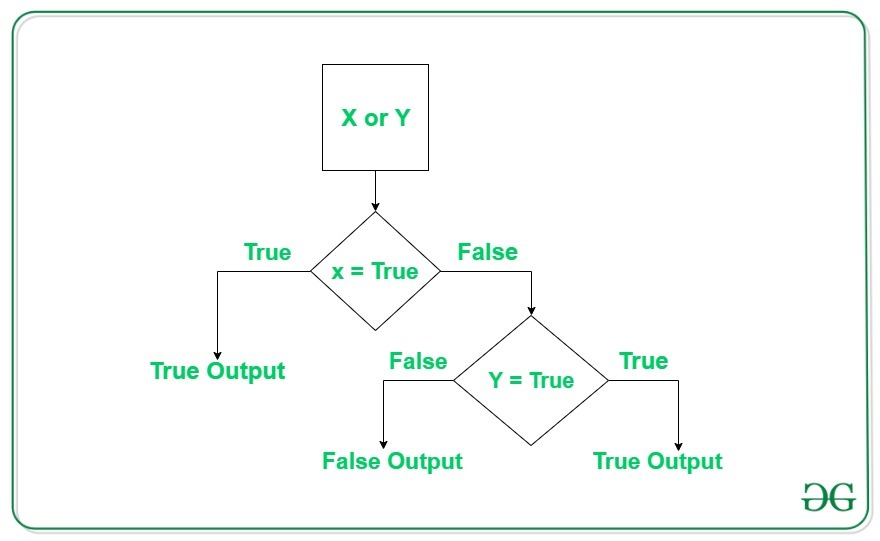Open in App
Not now

# Python OR Operator

• Last Updated : 19 Oct, 2021

Python OR Operator takes at least two boolean expressions and returns True if any one of the expressions is True. If all the expressions are False then it returns False.

## Flowchart of Python OR Operator## Using Python OR Operator with Boolean Expression

Python OR operator returns True in any one of the boolean expressions passed is True.

## Python3

 `bool1 ``=` `2``>``3``bool2 ``=` `2``<``3`` ` `print``(``'bool1:'``, bool1)``print``(``'bool2:'``, bool2)`` ` `# or operator``OR ``=` `bool1 ``or` `bool2``print``(``"OR operator:"``, OR)`

Output

```bool1: False
bool2: True
OR operator: True```

## Using Python OR Operator in if

We can use the OR operator in the if statement. We can use it in the case where we want to execute the if block if any one of the conditions becomes if True.

## Python3

 `# or operator with if``def` `fun(a):``    ``if` `a >``=` `5` `or` `a <``=` `15``:``        ``print``(``'a lies between 5 and 15'``)``    ``else``:``        ``print``(``'a is either less than 5 or greater than 15'``)`` ` ` ` `# driver code``fun(``10``)``fun(``20``)``fun(``5``)`

Output

```a lies between 5 and 15
a lies between 5 and 15
a lies between 5 and 15```

In the above output, we can see that the code for the if statement is executed always. This is because for every value of a, one of the boolean expressions will always be True and the else block will never get executed.

## Python OR Operator – Short Circuit

The Python Or operator always evaluates the expression until it finds a True and as soon it Found a True then the rest of the expression is not checked. Consider the below example for better understanding.

## Python3

 `# short circuit in Python or operator``def` `true():``    ``print``(``"Inside True"``)``    ``return` `True`` ` `def` `false():``    ``print``(``"Inside False"``)``    ``return` `False`` ` `case1 ``=` `true() ``or` `false()``print``(``"Case 1"``)``print``(case1)``print``()`` ` `case2 ``=` `true() ``or` `true()``print``(``"Case 2"``)``print``(case2)``print``()`` ` `case3 ``=` `false() ``or` `false()``print``(``"Case 3"``)``print``(case3)``print``()`` ` `case4 ``=` `false() ``or` `true()``print``(``"Case 4"``)``print``(case4)`

Output

```Inside True
Case 1
True

Inside True
Case 2
True

Inside False
Inside False
Case 3
False

Inside False
Inside True
Case 4
True```

From the above example, we can see that the short circuit or lazy evaluation is followed. In case1 and case2, the second expression is not evaluated because the first expression returns True, whereas, in case3 and case4 the second expression is evaluated as the first expression does not returns True.

My Personal Notes arrow_drop_up